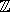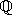## CGAL::Simple_homogeneous<RingNumberType>

#include <CGAL/Simple_homogeneous.h>

### Definition

A model for a Kernel using homogeneous coordinates to represent the geometric objects. In order for Simple_homogeneous<RingNumberType> to model Euclidean geometry in E2 and/or E3, for some mathematical ring E (e.g., the integersor the rationals), the template parameter RingNumberType must model the mathematical ring E. That is, the ring operations on this number type must compute the mathematically correct results. If the number type provided as a model for RingNumberType is only an approximation of a ring (such as the built-in type double), then the geometry provided by the kernel is only an approximation of Euclidean geometry.

Kernel

### Types

 typedef Quotient FT; typedef RingNumberType RT;

### Implementation

In contrast to Homogeneous, no reference counting is used internally. This eases debugging, but may slow down algorithms that copy objects intensively, or slightly speed up others.